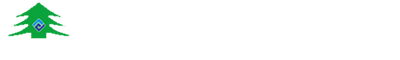• 1
• 2
• 3
•••0393-5562939 工作时间：周一至周五
8:00-18:00

18103938983

# 您的位置：首页 >> 新闻中心

1、钢瓶内容积V = 40 L

2、压缩气体的充气压力P =12.6 MPa（绝对压力)

3、汇流排自动切换压力P1 =1.1MPa （绝对压力）

4、不考虑管路的容积；

5、气体体积变化时温度不变。

V1=VP/P1–V

V0=V1P1/P0

P0 —— 标准大气压，绝对压力近似为 0.1 MPa

V0 —— 压力为标准大气压的可用气体容积（L）。

V1 = 40×12.6/1.1-40=418.18L

V0 = 418.18×1.1/0.1=4600 L

1、钢瓶内容积V=40L

2、充装量18Kg

3、汇流排自动切换压力P1=1.1MPa（绝对压力）；

4、不考虑管路的容积；

5、气体体积变化时温度不变。

8Kg液态二氧化碳汽化生成的标准气体体积：

V3=18000/1.977=9105L

V4=VP1/P0=40×1.1/0.1=440L

V0=V3-V4=8665L

1、钢瓶内容积V=40L

2、充装量20Kg

3、汇流排自动切换压力P1=1.1MPa（绝对压力）；

4、不考虑管路的容积；

5、气体体积变化时温度不变。

20Kg液态笑气汽化生成的标准气体体积：

V5=20000/1.978=10111L

V6=VP1/P0=40×1.1/0.1=440L

V0=V5-V6=9671L

1、压力为0.15MPa的可用氧气体积为

V1.5=40×12.6/0.25-40=1976L

2、压力为常压（绝对压力0.1MPa）的可用氧气体积为

V0=V1.5×0.25/0.1=4940L=4.94m3

3、可用氧气的重量为G=1.429×V0=7Kg）。

1、绝对压力为0.7MPa的可用氮气体积为V0.6=40×12.6/0.7-40=680L

2、压力为常压（大气压）的可用氮气体积为 V0=V0.6×0.7/0.1=4760L=4.76m3

3、可用氮气的重量为G=1.251×V0=6Kg）。1、氧气的换算